# 圖資料探勘！使用圖分析+AI進行保險欺詐檢測

## highlight: a11y-dark• Graph 圖與圖挖掘基礎
• 圖搜尋和查詢以瞭解關係
• 使用圖特徵增強機器學習模型

# 💡 Graph圖與圖挖掘基礎

## 📌 什麼是Graph圖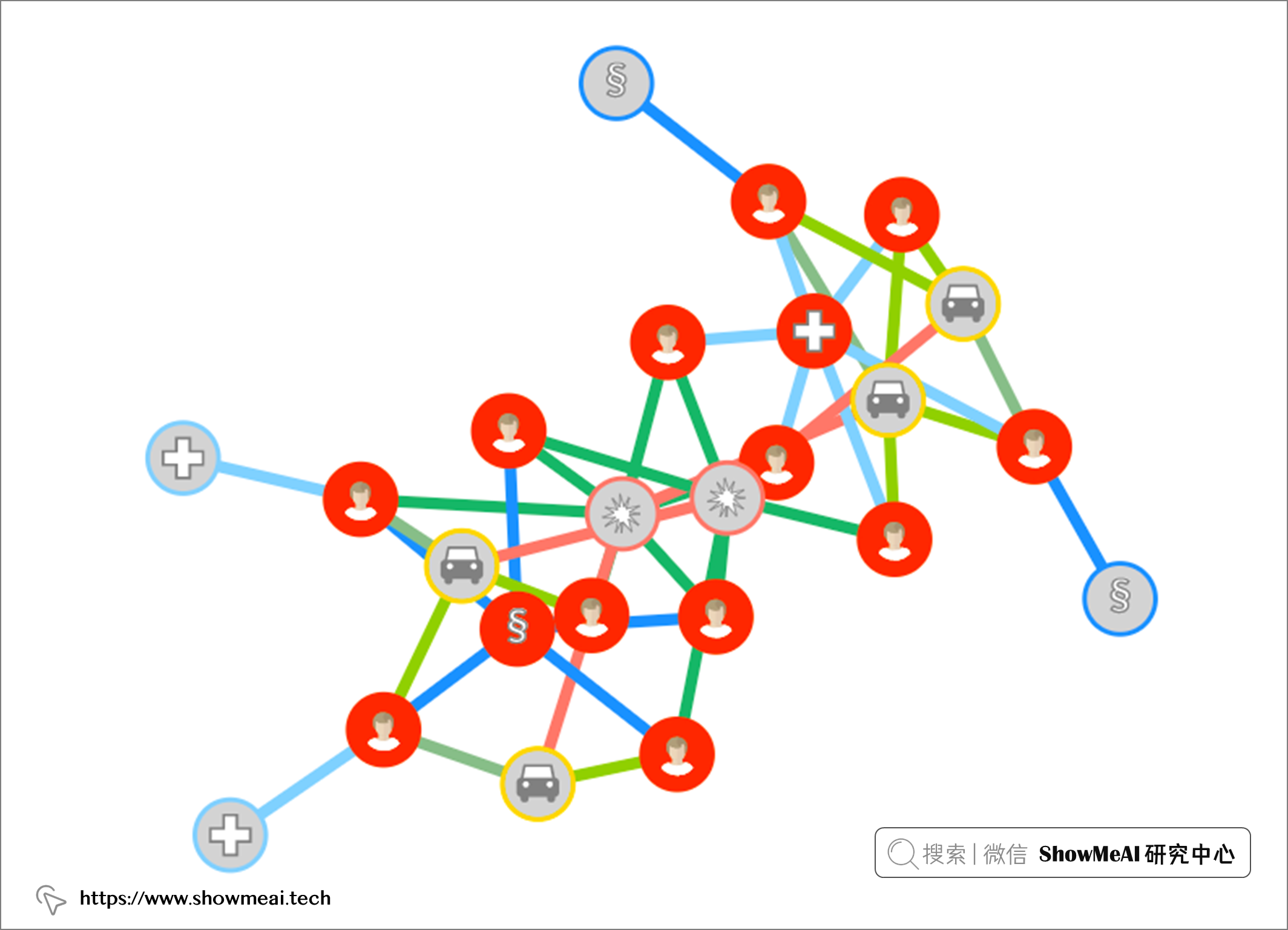• 節點，在醫療保險場景中，可以是
• 投保人
• 醫生
• 邊是節點之間的關係/互動/行為
• 索賠保單持有人
• 索賠被保險人

# 💡 圖挖掘：搜尋和查詢

🏆 實戰資料集下載（百度網盤）：公眾號『ShowMeAI研究中心』回覆『實戰』，或者點選 這裡 獲取本文 圖資料探勘！使用圖分析+AI進行保險欺詐檢測insurance claims 保險索賠資料集

ShowMeAI官方GitHubhttps://github.com/ShowMeAI-Hub

## 📌 欺詐典型案例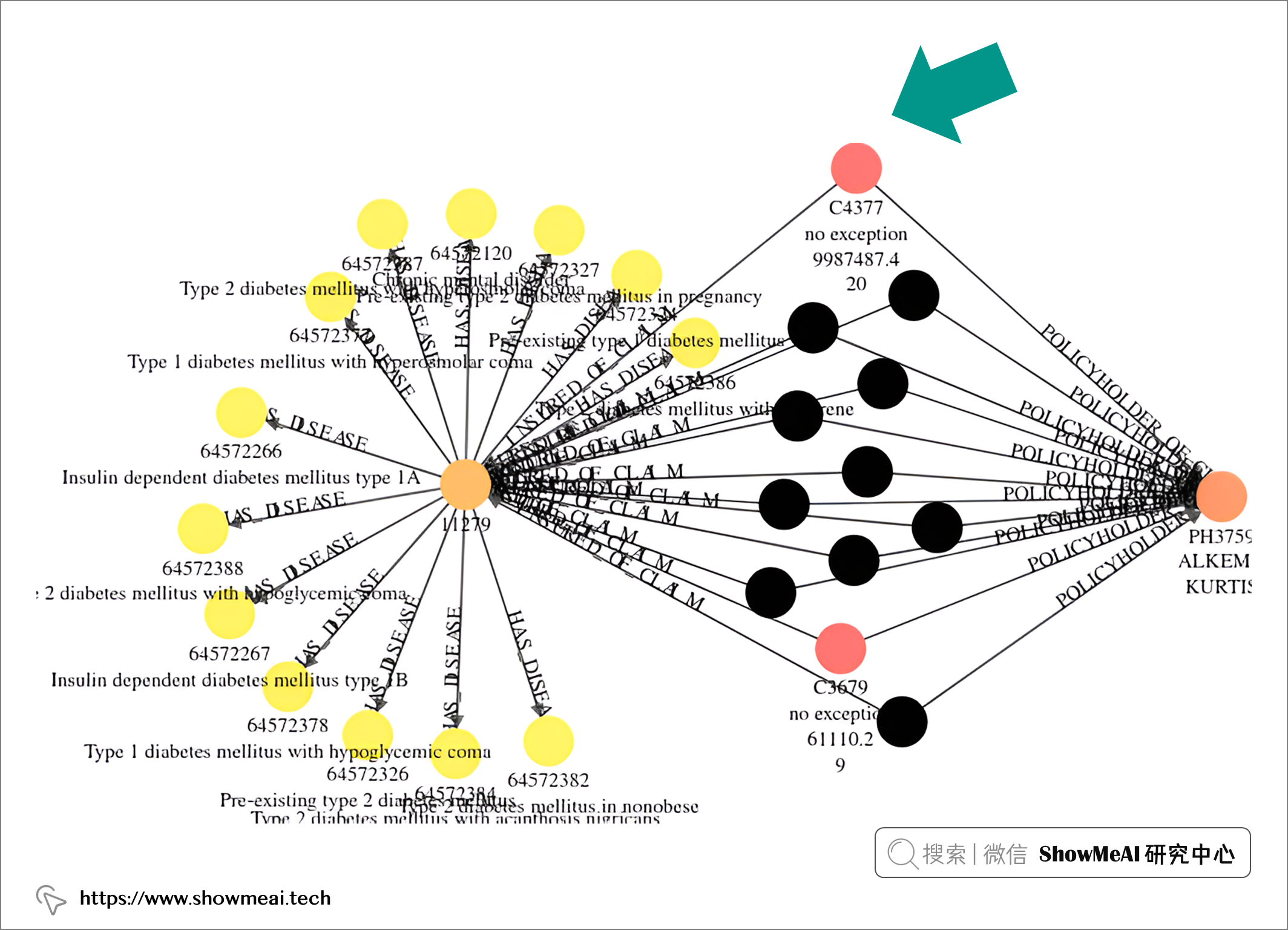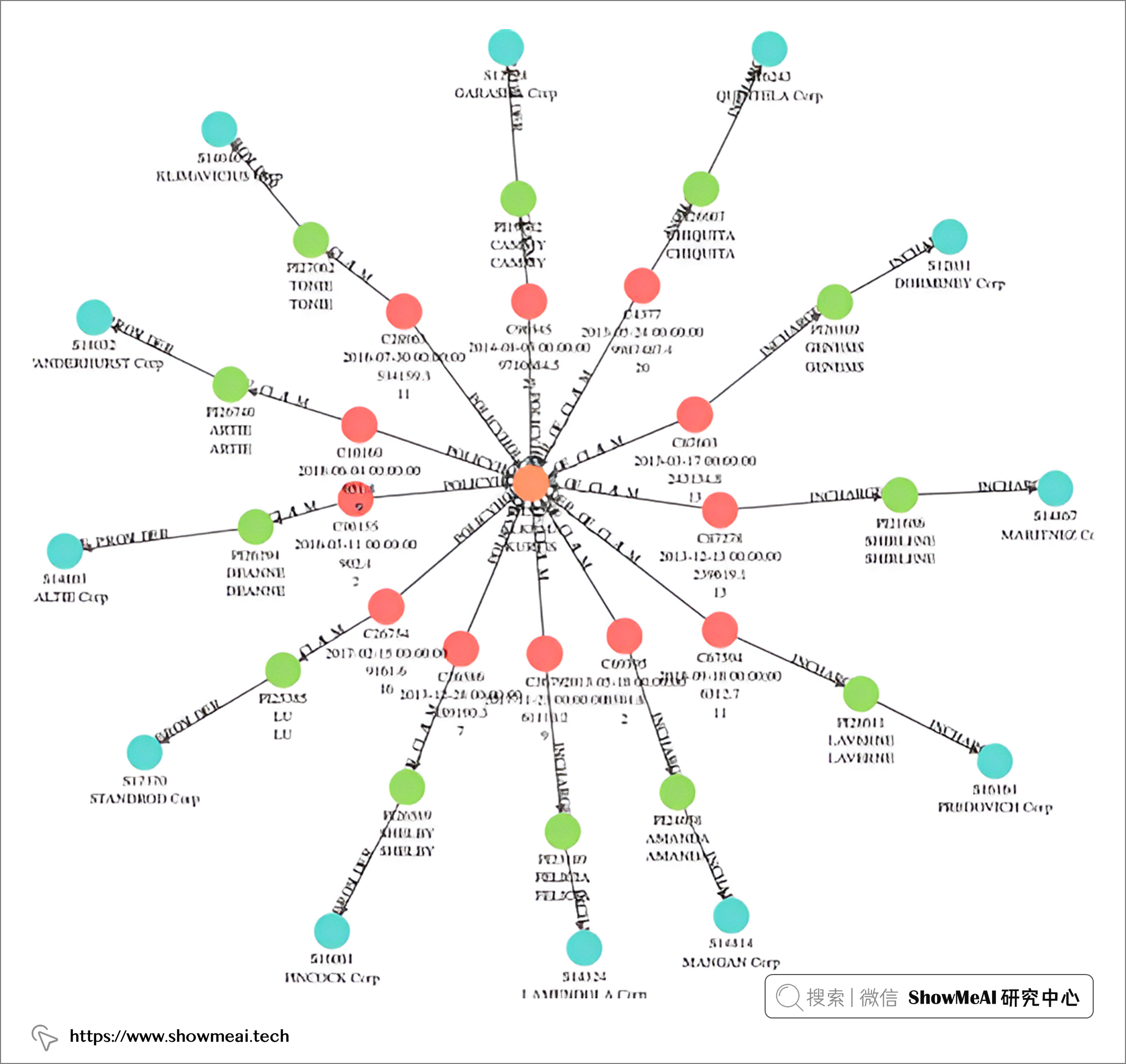## 📌 與欺詐性索賠相關的投保人關係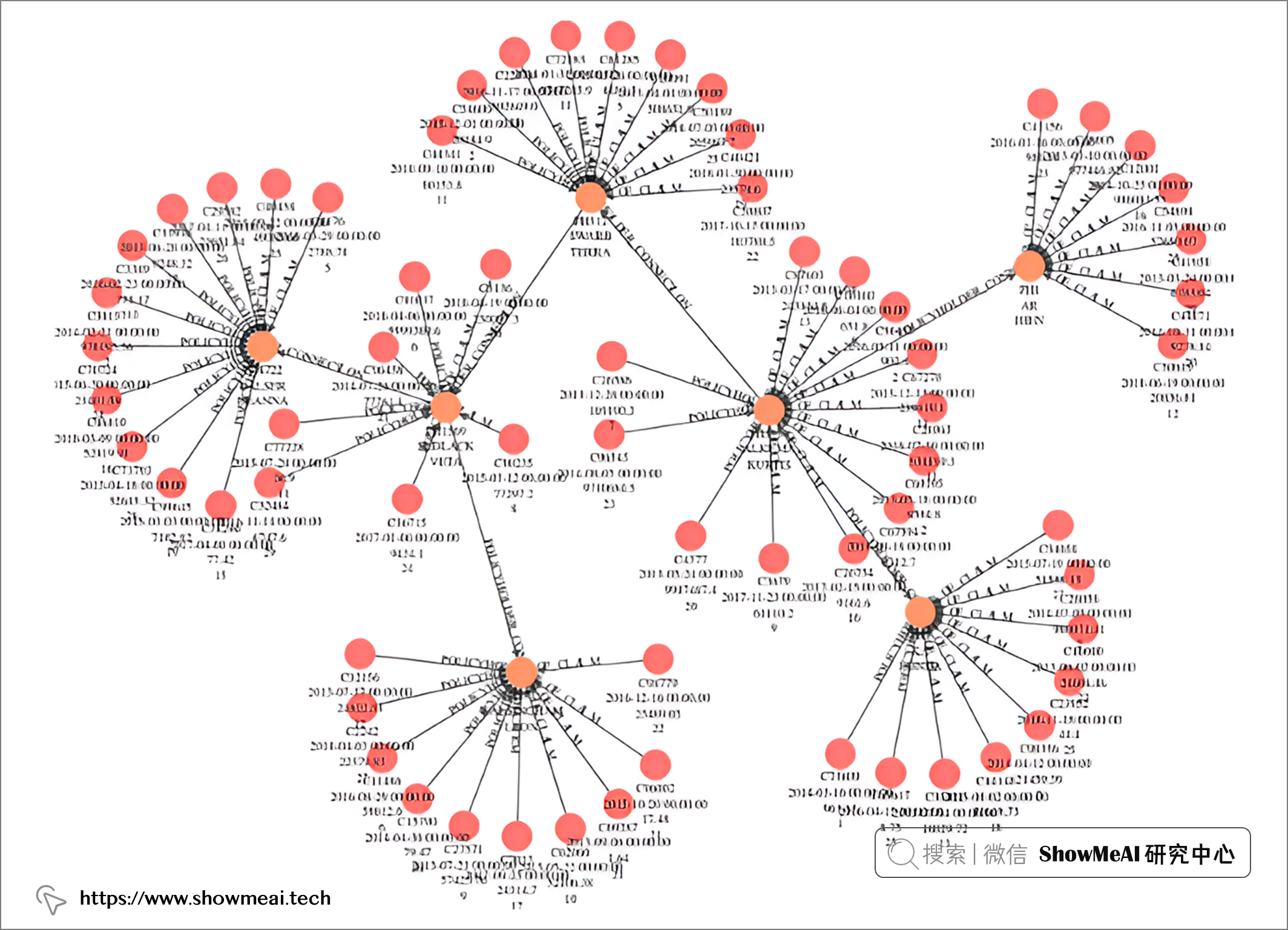# 💡 使用圖特徵增強機器學習模型

• 將結構化資料提取到 Python 圖結構中
• 圖資料的特徵工程
• 將圖特徵整合到機器學習管道中

## 📌 將結構化資料提取到圖結構中

🏆 實戰資料集下載（百度網盤）：公眾號『ShowMeAI研究中心』回覆『實戰』，或者點選 這裡 獲取本文 圖資料探勘！使用圖分析+AI進行保險欺詐檢測insurance claims 保險索賠資料集

ShowMeAI官方GitHubhttps://github.com/ShowMeAI-Hub

• 患者相關特徵：年齡、性別、位置、健康狀況等。
• 索賠相關特徵：起止日期、索賠金額、診斷程式碼、程式程式碼、提供者、主治醫師、手術醫師等。

```python

# 匯入包

from igraph import * import pandas as pd

# 讀取資料

df = pd.read_csv('CLAIM.csv') G_df = df[['CLAIM_ID', 'POLICYHOLDER_ID']]

# 從Dataframe建立Graph無向圖

G = Graph.DataFrame(G_df, directed=False) ```

## 📌 圖資料特徵工程

### Degree 度

```python degree = pd.DataFrame({'Node': G.vs["name"], 'Degree': G.strength()}) degree.shape```

### closeness 接近中心性

```python closeness = pd.DataFrame({'Node': G.vs["name"], 'Closeness': G.closeness()}) closeness.shape```

### Infomap 資訊圖

Infomap是一種能夠實現高質量社群的圖聚類演算法。

```python communities_infomap = pd.DataFrame({'Node': G.vs["name"], 'communities_infomap': G.community_infomap().membership}) communities_infomap.shape```

```python

# 合併圖特徵

graph_feature = [degree, closeness, communities_infomap] graph_feature = reduce(lambda left,right: pd.merge(left,right, how = 'left',on='Node'), graph_feature) ```

## 📌 將圖特徵整合到機器學習管道中

```python train = Final_Dataset_Train[Final_Dataset_Train['ClaimStartDt'] < '2009-10-01'].reset_index(drop = True).drop('ClaimStartDt', axis = 1) print(train.shape) test = Final_Dataset_Train[Final_Dataset_Train['ClaimStartDt'] >= '2009-10-01'].reset_index(drop = True).drop('ClaimStartDt', axis = 1) print(test.shape)x_tr = train.drop(axis=1,columns=['PotentialFraud']) y_tr = train['PotentialFraud']x_val = test.drop(axis=1,columns=['PotentialFraud']) y_val = test['PotentialFraud']```

```python

# 建模示例程式碼

lr = LogisticRegression(penalty='none', solver='saga', random_state=42, n_jobs=-1) rf = RandomForestClassifier(n_estimators=300, max_depth=5, min_samples_leaf=50, max_features=0.3, random_state=42, n_jobs=-1) lr.fit(x_tr, y_tr) rf.fit(x_tr, y_tr) preds_lr = lr.predict_proba(x_val)[:,1] preds_rf = rf.predict_proba(x_val)[:,1] ```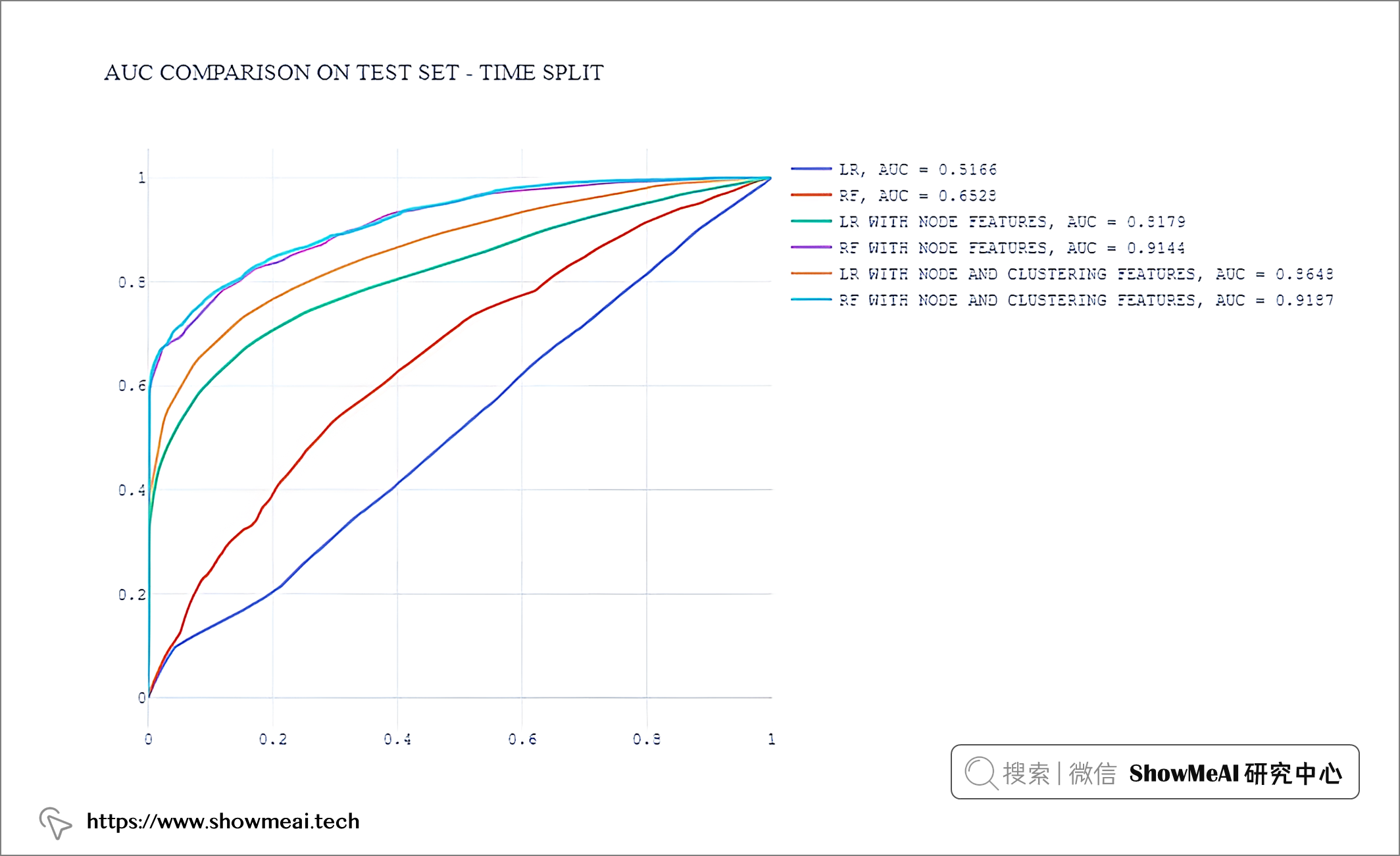• 具有圖特徵的模型表現出色
• 節點級別特徵效果非常好
• 聚類特徵對結果也有補充作用

# 參考資料